## Introduction

Have you ever wanted to apply an Excel function in your VBA code? Have you ever wanted to write a macro but spent hours trying to find a built-in VBA VLookup Function?

There are many built-in Excel functions that aren’t available in VBA, but don’t give up. Use Application.WorksheetFunction to call VLookup, Max, Min and many more Excel functions directly from your VBA macro. This tutorial will show you how.

## VBA VLookup

Let’s say you have the following data in an Excel spreadsheet and you want to use standard Excel functions to analyze the data: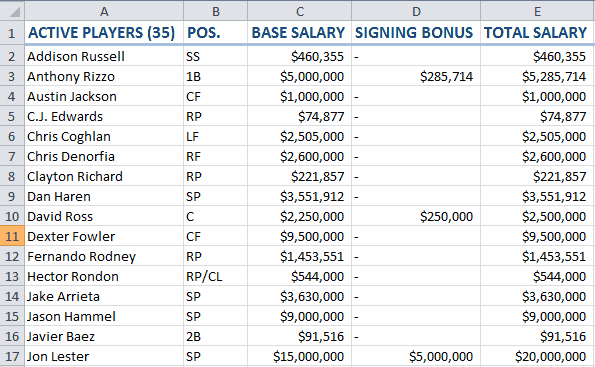In this VBA example, we want to use VLookup to tell us the total salary of Chicago Cubs pitcher, Jake Arrieta. Here’s how you would do it with a macro:

``````Sub VLookupFunctionDemo()
Dim d1 As Double
d1 = Application.WorksheetFunction.VLookup("Jake Arrieta", Range("A2:E36"), 5)
MsgBox Format(d1, "Currency"), , "VBA VLookup Function"
End Sub``````

The macro produces the following results: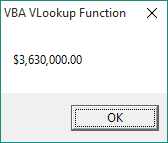VBA VLookup Results

Make powerful macros with our free VBA Developer Kit

It’s easy to copy and paste a macro like this, but it’s harder make one on your own. To help you make macros like this, we built a free VBA Developer Kit and wrote the Big Book of Excel VBA Macros full of hundreds of pre-built macros to help you master file I/O, arrays, strings and more - grab your free copy below.

I'll take a free VBA Developer Kit

You’ll notice that when you start typing `VLookup(`, a Quick Info box will appear, but all it shows is Arg1, Arg2, Arg3, [Arg4].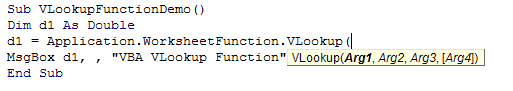VBA VLookup Arguments

This isn’t very useful, but just know that the arguments of WorksheetFunction functions are identical to the arguments of the equivalent standard Excel functions. The syntax, however, may be different, since you’ll be programming in VBA instead of Excel.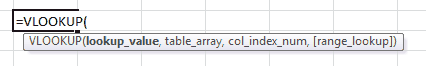Excel VLOOKUP Arguments

Rest assured, if you know how to use the formula in Excel, you’ll know how to use the formula using the VBA WorksheetFunction method. If you don’t know how to use the VLookup formula in Excel, I encourage you to check out this awesome tutorial from my friend at Spreadsheeto.

## VBA Max

Believe it or not, there’s no exclusive VBA Max function! This shocked me when I was first learning VBA because I came from a FORTRAN background. The nuclear industry is old, so don’t make fun of my FORTRAN background! People argue FORTRAN is a primitive programming language, but even it has a native maximum function.

All is not lost. By using the WorksheetFunction method, you can still call the standard Excel Max function within your VBA macro. Here’s an example:

``````Sub MaxFunctionDemo()
Dim d1 As Double
d1 = Application.WorksheetFunction.Max(Range("E1:E36"))
MsgBox d1, , "VBA Max Function"
End Sub``````

## VBA Min

Another example, but this time with the Excel Min function.

``````Sub MinFunctionDemo()
Dim d1 As Double
d1 = Application.WorksheetFunction.Min(Range("E2:A36"))
MsgBox d1, , "VBA Min Function"
End Sub``````

If you dig back in the archives, you’ll notice I use both the Excel Max and Min functions in my comparing two columns article. They truly are some of the most useful worksheet functions.

## Closing Remarks

I’ve only touched on a few of the dozens of functions you can call using Application.WorksheetFunction. For a complete list, open your VBA Editor and begin typing `Application.WorksheetFunction.`. If you have “Complete Word” enabled (Ctrl-Space), you’ll be able to scroll through dozens of built-in Excel functions that you can use in your VBA macros!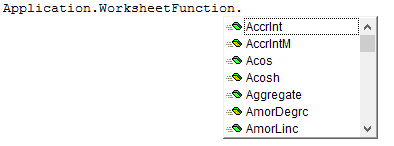VBA WorksheetFunction Functions

That’s all for this tutorial. When you’re ready to take your VBA to the next level, subscribe using the form below.